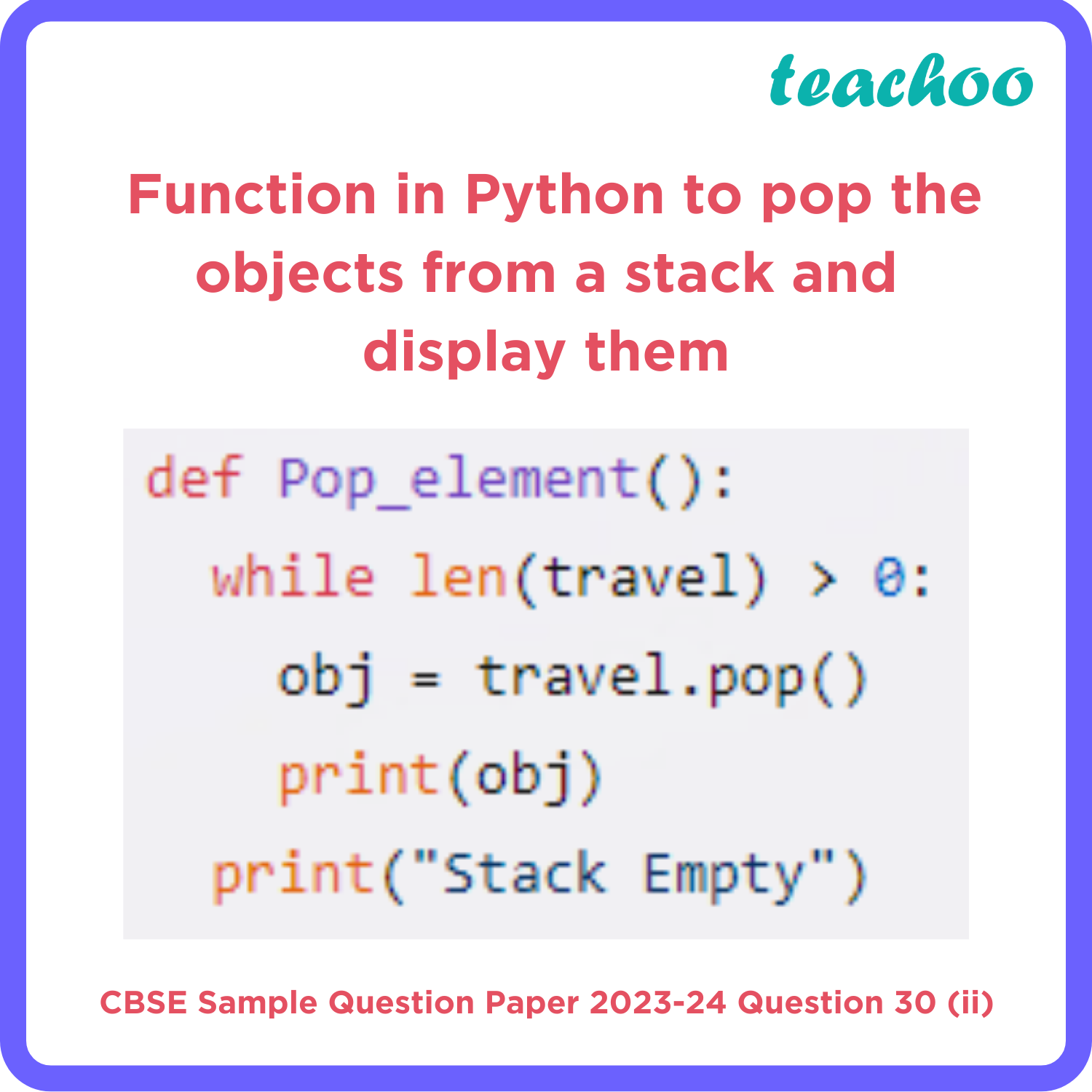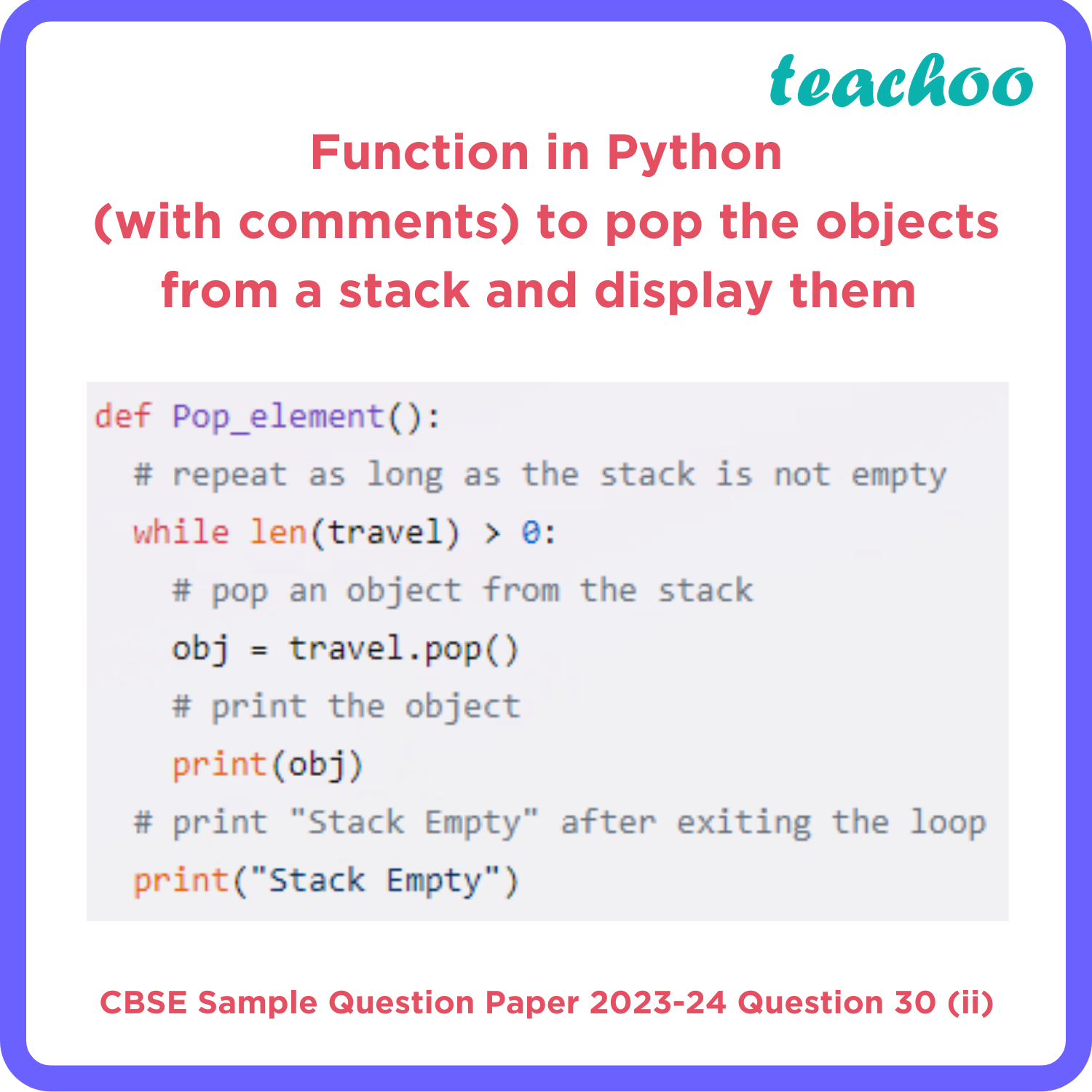CBSE Class 12 Sample Paper for 2024 Boards

Computer Science - Class 12
Solutions to CBSE Sample Paper - Computer Science Class 12

## Stack Empty• The question asks us to write a user defined function in Python to perform the specified operations on the stack named travel, which is a list that can be manipulated using the pop() method. The pop() method removes and returns the last element of the list.
• The function should pop the objects from the stack and display them. Also, the function should display “Stack Empty” when there are no elements in the stack.
• To do this, we need to use the following steps:
• Define the function using the def keyword, followed by the function name and parentheses. In this case, we name the function Pop_element and use no parameters.
• Use a while loop to repeat a block of code as long as a condition is True. The loop will end when the condition becomes False, which means that the stack is empty.
• Pop an object from the stack using the pop() method, which removes and returns the last element of the list. For example, travel.pop() will return and remove the last element of the travel list. We assign this value to a variable named obj.
• Print the value of obj using the print() function, which displays the value of its argument to the standard output.
• Print “Stack Empty” using the print() function after exiting the loop.
• End the function definition with proper indentation.